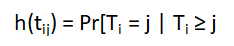# What Is a Hazard Function in Survival Analysis?

One of the key concepts in Survival Analysis is the Hazard Function.

But like a lot of concepts in Survival Analysis, the concept of “hazard” is similar, but not exactly the same as, its meaning in everyday English. Since it’s so important, though, let’s take a look.

### Hazard: What is It?

If you’re not familiar with Survival Analysis, it’s a set of statistical methods for modelling the time until an event occurs.

Let’s use an example you’re probably familiar with — the time until a PhD candidate completes their dissertation.

Each person in the data set must be eligible for the event to occur and we must have a clear starting time. So a good choice would be to include only students who have advanced to candidacy (in other words, they’ve passed all their qualifying exams).

​​​​​​​Likewise we have to know the date of advancement for each student. This date will be time 0 for each student.

The hazard is the probability of the event occurring during any given time point. It is easier to understand if time is measured discretely, so let’s start there.

Let’s say that for whatever reason, it makes sense to think of time in discrete years. For example, it may not be important if a student finishes 2 or 2.25 years after advancing. Practically they’re the same since the student will still graduate in that year.

So for each student, we mark whether they’ve experienced the event in each of the 7 years after advancing to candidacy. Of course, once a student finishes, they are no longer included in the sample of candidates.

We can then calculate the probability that any given student will finish in each year that they’re eligible. That’s the hazard.

In fact we can plot it. Below we see that the hazard is pretty low in years 1, 2, and 5, and pretty high in years 4, 6, and 7.

​​​​​​​We can then fit models to predict these hazards. For example, perhaps the trajectory of hazards is different depending on whether the student is in the sciences or humanities.### Calculating a Hazard

But where do these hazards come from? Let’s look at an example.

Let’s say we have 500 graduate students in our sample and (amazingly), 15 of them (3%) manage to finish their dissertation in the first year after advancing.

Our first year hazard, the probability of finishing within one year of advancement, is .03. That is the number who finished (the event occurred)/the number who were eligible to finish (the number at risk).

In the first year, that’s 15/500. 15 finished out of the 500 who were eligible.

Now let’s say that in the second year 23 more students manage to finish. The second year hazard is 23/485 = .048. You’ll notice this denominator is smaller than the first, since the 15 people who finished in year 1 are no longer in the group who is “at risk.”

All this is summarized in an intimidating formula:All it says is that the hazard is the probability that the event occurs during a specific time point (called j), given that it hasn’t already occurred.

### Why hazard? That sounds so ominous.

Yeah, it’s a relic of the fact that in early applications, the event was often death. So a probability of the event was called “hazard.”

It feels strange to think of the hazard of a positive outcome, like finishing your dissertation. But technically, it’s the same thing.

### When Time is Continuous

The concept is the same when time is continuous, but the math isn’t. If time is truly continuous and we treat it that way, then the hazard is the probability of the event occurring at any given instant.

If you’re familiar with calculus, you know where I’m going with this. Because there are an infinite number of instants, the probability of the event at any particular one of them is 0.

​​​​​​​​​​​​​​That’s why in Cox Regression models, the equations get a bit more complicated. Here we start to plot the cumulative hazard, which is over an interval of time rather than at a single instant.Introduction to Survival Analysis
Learn the key tools necessary to learn Survival Analysis in this brief introduction to censoring, graphing, and tests used in analyzing time-to-event data.